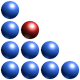# Complexity Is ….. Not That Simple

A QCM (Quantitative Complexity Management) analysis of a data set produces what is known as a Complexity Map – an illustration of the topology of information flow within a given system. An example is shown below.

The discs are known as hubs and they indicate variables that have a larger footprint on the entire system (i.e. data set under analysis). This looks pretty stratightforward. Let’s also forget for a moment that the synthesis of the map involves a sophisticated mechanism for computing the so-called generalized correlations, which replace the popular and often risky linear (Pearson’s) correlations.

Once the Complexity Map is avaliable, the complexity of a given system may be computed, as well as its bounds, especially the upper bound known as critical complexity. So far so good.

However, what is not so obvious is that the Complexity Map is only the tip of the iceberg when it comes to understanding complexity and its structure. Complexity is not just a number that is attached to a graph, i.e. the Complexity Map. In fact, when (multi-dimensional) data is ‘rugged’, the Complexity Map is a topological sum of other ‘smaller’ complexity maps known as modes. An example is illustrated below:

What most users of our QCM tools get to see is what is on the left hand side of the equation. Real, deep understanding of complexity means knowing what is going on on the right hand side too. Each complexity mode (map) has its own complexity as well as ‘intensity’, which is measured with its frequency of appearance within a given data set. We could refer to this frequency as probability.

The Complexity Spectrum of a system is graph wich ranks complexity modes according to their complexity, see example below:

The modal intensity ‘ modal ‘strength’ chart is similar:

It is clear how modes 1, 5, 7, 10 and 18 in this case are the ‘dominant’ modes. It is these modes that drive complexity. The remaining modes are called residual modes. OntoSpace, our flagship QCM product, arranges modes in a tree as shown below:

The order of modes in the tree is according to their respective ‘strenght’, i.e. probability of occurrence. The more rugged the underlying data is the more complexity modes there are. This kind of analysis represents what happens at one single time step. Imagine now how involved things can get when the above is repeated at each step of analysis.

This very short blog illustrates why high complexity is a problem. The dynamics of very complex systems can be extremely involved since their behaviour is driven by dominant modes which can change over time. Mode switching can often happen spontaneously, or due to even minute changes in certain underlying parameters. And herein lies the problem. Mode switching means the system suddenly starts to behave differently. It starts to suddenly show a different face, sometimes developing chaos or other forms of intricate behaviour, peppered with bifurcations or attractors. Very high complexity means this happens more frequently and the degree of surprise increases. This can make control of such systems very difficult. A small example of what this means is shown below.

The above is a very small case with only 120 data channels (variables).

The above hopefully provides a simple explanation as to why it is dangerous to fiddle with highly complex systems. Some examples of super complex systems with which people like to fiddle are:

• the DNA
• the human immune system
• society
• the global financial system
• human nature

Understanding the nature and dynamics of these systems poses a major challenge not just to science but to politicians and to humanity as a whole. More soon.

www.ontonix.com